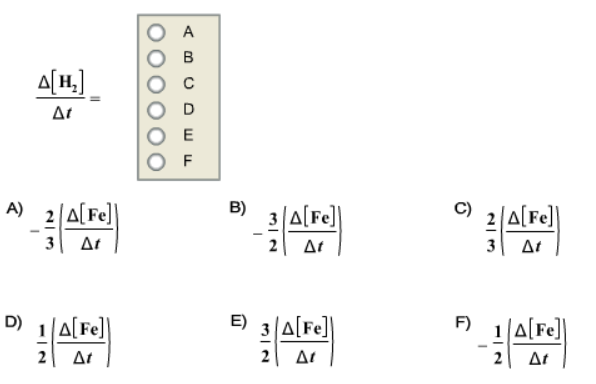# Problem: Given the following balanced chemical equation2Fe+3H2O → Fe2O3+3H2 How is the rate of appearance of H2 related to the rate of disappearance of Fe?

###### FREE Expert Solution

Recall that the average rate of a reaction is given by:where Δ[A] = change in concentration of reactants or products (in mol/L or M) and Δtime = change in time. We can simply do a rate-to-rate comparison based on the stoichiometric coefficients.###### Problem Details

Given the following balanced chemical equation

2Fe+3H2O → Fe2O3+3H2

How is the rate of appearance of H2 related to the rate of disappearance of Fe?